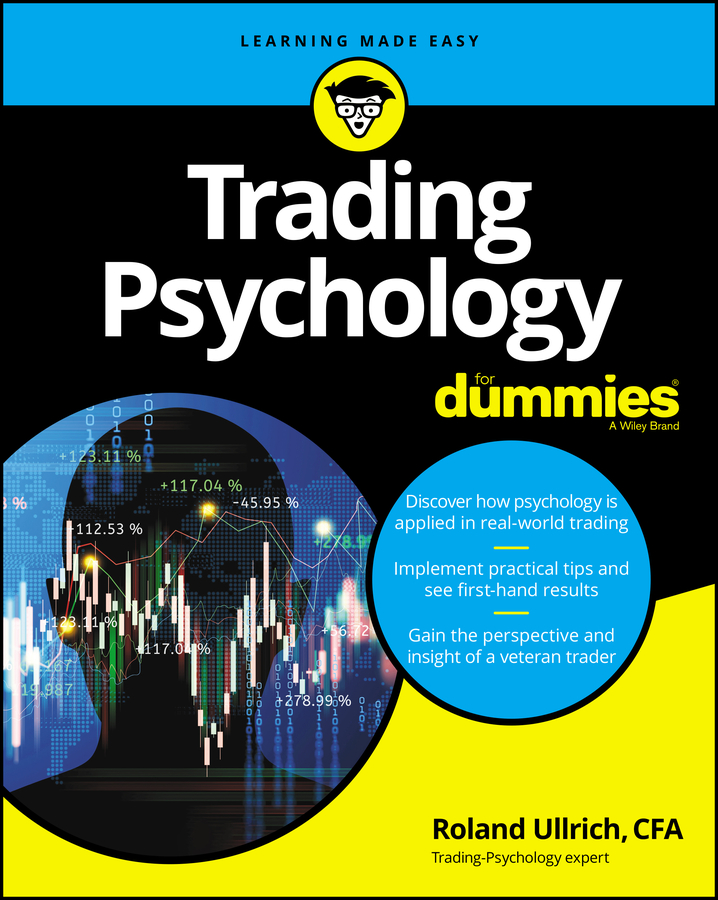Over the years, day traders have developed many different ways to manage their money. Some of these are rooted in superstition, but most are based on different statistical probability theories. The underlying idea is that you should never place all of your money in a single trade, but rather put in an amount that is appropriate given the level of volatility. Otherwise, you risk losing everything.

Calculating position size under many of these formulas is tricky stuff. That’s why brokerage firms and trading software packages often include money management calculators.

## Fixed fractional

Fixed fractional trading assumes that you want to limit each trade to a set portion of your total account, often between 2 and 10 percent. Within that range, you’d trade a larger percentage of money in less risky trades and at the smaller end of the scale for more risky trades.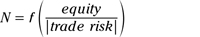The equation for calculating fixed fractional trade proportions.

N is the number of contracts or shares of stock you should trade, f is the fixed fraction of your account that you have decided to trade, equity is the value of your total account, and trade risk is the amount of money you could lose on the transaction. Because trade risk is a negative number, you need to convert it to a positive number (absolute value) to make the equation work.

This means that if you have decided to limit each trade to 10% of your account, if you have a \$20,000 account, and if the risk of loss is –\$3,500, your trade should be as follows: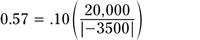An example of a fixed fractional trade calculation.

Of course, you probably can’t trade .57 of a contract, so in this case, you would have to round up to one.

## Fixed ratio

The fixed ratio money management system is used in trading options and futures. The idea behind fixed ratio trading is to help you increase your exposure to the market while protecting your accumulated profits. In order to find the optimal number of options or futures contracts to trade, N, you use this equation: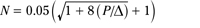The equation for calculating fixed ratio trading proportions.

P is your accumulated profit to date, and the triangle, delta, is the dollar amount that you would need before you could trade a second contract or another lot of stock. (This is not the same delta that is a measure of volatility.)

For example, the minimum margin for a Chicago Mercantile Exchange E-Mini S&P 500 futures contract is \$3,500. Until you have another \$3,500 in your account, you can’t trade a second contract. If you're using fixed ratio money management to trade this future, your delta will be \$3,500.

If your delta is \$3,500, and you have \$10,000 in account profits, you should trade 1.2 contracts (see figure). In reality, that means you can only trade one contract or two contracts, nothing in between. That’s one of the imperfections of most money management systems.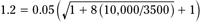An example using the fixed ratio calculation.